Function Repository Resource:

# ThieleExpansion

Expand a function into a Thiele continued fraction

Contributed by: Jan Mangaldan
 ResourceFunction["ThieleExpansion"][f,{x,x0,n}] generates a Thiele continued fraction expansion for f about the point x=x0 up to the nth order convergent, where n is an explicit integer.

## Details and Options

ResourceFunction["ThieleExpansion"] can find the Thiele continued fraction expansion about the point x=x0 only when it can evaluate power series at that point.
ResourceFunction["ThieleExpansion"] takes the following option:
 Method Automatic method to use
Possible settings for Method include "Viscovatov" and "WynnOmega", with the default being Automatic.

## Examples

### Basic Examples (2)

Compute the Thiele continued fraction for the exponential function around x=0:

 In:=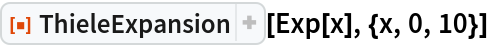Out=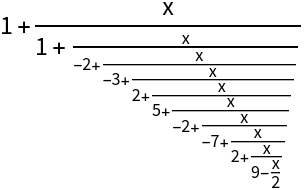Thiele continued fraction of an arbitrary function around x=a:

 In:=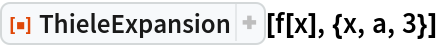Out=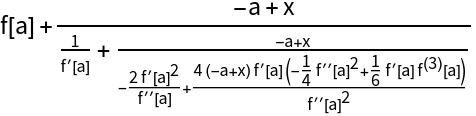### Options (2)

#### Method (2)

Use different methods for generating the Thiele expansion:

 In:=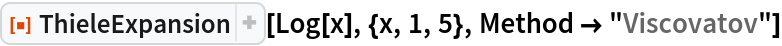Out=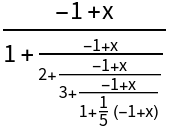In:=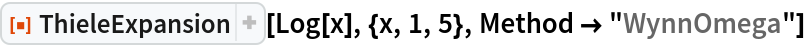Out=The two methods will give results that are mathematically equivalent but may differ in form:

 In:=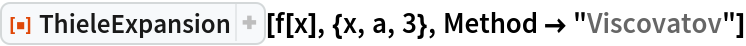Out=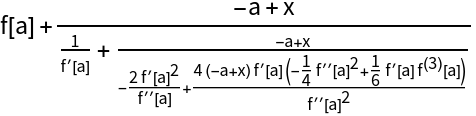In:=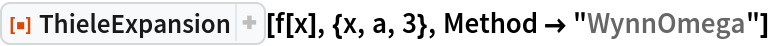Out=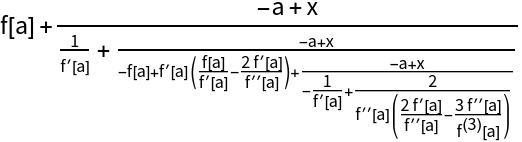### Applications (1)

Plot successive Thiele continued fraction approximations to exp(x):

 In:=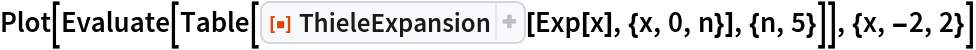Out=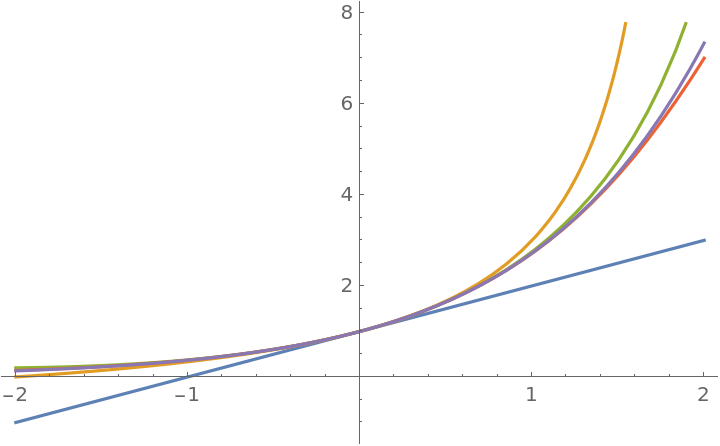### Properties and Relations (1)

The Thiele expansion of order n is equivalent to the result of PadeApproximant of order {Ceiling[n/2],Floor[n/2]}:

 In:=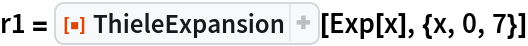Out=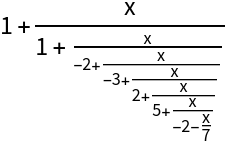In:=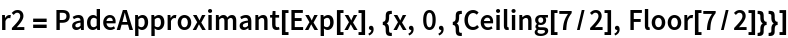Out=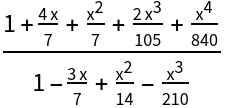In:=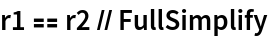Out=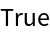### Possible Issues (2)

Thiele expansion is not defined for even or odd functions:

 In:=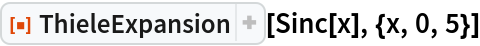Out=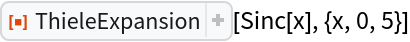Transform the variable to get a continued fraction expansion:

 In:=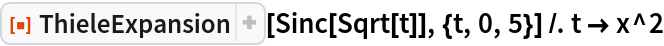Out=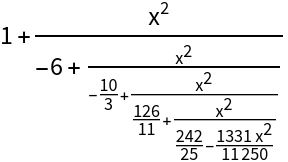## Version History

• 1.0.0 – 13 January 2021